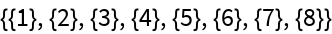#Function Repository Resource:

# FindCanonicalHypergraph

Find a canonical version of a directed hypergraph

Contributed by: Ed Pegg Jr
 ResourceFunction["FindCanonicalHypergraph"][graph] finds a canonical version of a hypergraph.

## Details and Options

The canonicalization follows several rules:
 1 Tuples are sorted by length with longest tuples occuring first. 2 Tuples of the same length are considered a rule part. 3 A rule part with 3 tuples of length 2 has signature 32. 4 Rule parts are ordered so that new alphabet terms are introduced with maximal frugality.
ResourceFunction["FindCanonicalHypergraph"] uses a heuristic method and may not give a complete canonical form in all cases.

## Examples

### Basic Examples

Find a canonical version of a very simple hypergraph:

 In:=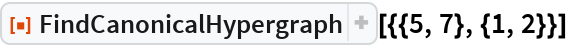Out=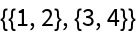A more complex case:

 In:=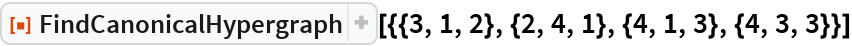Out=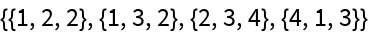Hyperedges are sorted by reverse length:

 In:=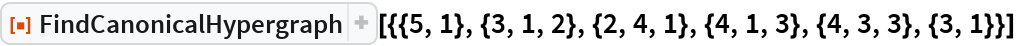Out=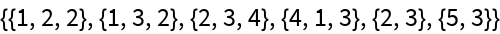### Possible Issues

Hypergraphs with many edges and extreme symmetry will take a while:

 In:=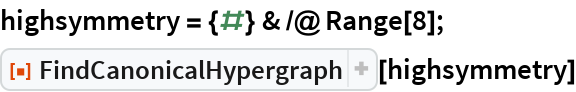Out=